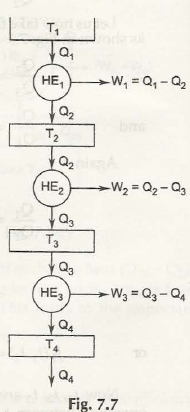According to the 1st law if there are more engines added to the below figure, total output will still not exceed Q1.Points to be noted:

• In some limited cases when you will get total output with equal amount as Q1 then heat rejection of the sink will reach zero.
• In other words it violates the rule of Kelvin Plank’s 2nd law which shows that there is some heat rejected at least to sink.
• In the limited case when heat sinking and heat rejection both are zero.
• Actually in most of the cases, no matter how many engines are added heat sinking will decrease but it will never reach zero.

We can now consider some examples with solutions to grab this matter completely.

Example: 1.A heat pump (HP), a heat engine (HE). The heat pump is determined by heat engine. The work out from HE is also driving one machine giving 30KW work input. Find.

(a) the rate of supply of heat from the 840°C source i.e., Q1E = ?

(b) the rate of rejection of total heat to 60°C heat reservoir (sink).

i.e., QlP + Q2E = ?

Solution:

ɳ HP = Q1P – Q2P/ Q1P = Q1P – 17 / Q1P

or1 – 273 + 5 / 273 + 60 = Q1P – 17 / Q1P

Q1P = 20.36 KW

[W = Q1P x ɳ HP = 20.36 x 0.165 = 3.36 kw]

W net for HE = 30 + W = 30 + 3.36

= 33.36 KW

For, HE,1 –Q2E /Q1E = 1 – 60 + 273 / 840 + 273

or Q2E / Q1E = 0.3

again Wnet= Q1E – Q2E

33.36 = Q1E – Q2E

Solving equations {1) and (2) we get Q1E = 47.66KW. Ans. (a)

Q2E = 0.3 x 47.66 = 14.3 KW

Thus total heat rejection to sink at 60°C

= QIP + Q2E = (20.36 + 14.3) KW

= 34.66 KW. Ans. (b)

Example 2: A heat engine is basically used to drive a heat pump. The heat transmits from the heat engine and from the heat pump which is actually is used to heat water mixing through the radiators of thestructure. The thermal efficacy of the heat engine is 30% and the heat pump is having the COP of 5%. Evaluate the ratio of the total heat transfers to the mixing water to the heat input to heat engine.

Solution.

ɳ HE = 0.3

1 – Q2E / Q1E = 0.3

Q2E / Q1E = 0.7

COP HP = Q1P / Q1P – Q2P =5

Q2P / Q1P = 1/5

1- Q2P / Q1P = 1/5

1 – 1/5 = Q2P / Q1P , Q2P / Q1P = 4 /5

We also have

W = Q1E  – Q2E = Q1P – Q2P

Q1E – Q1E x 0.7 = Q1P – 4 /5 Q1P

0.3 Q1E = Q1P /5

Q1P / Q1E = 1.5

To find Q2E + Q1P / Q1E = Q2E / Q1E + Q1P / Q1E = 0.7 + 1.5

Thus the radio is 2.2.

Example 3: If 30 KJ are added to Car not cycle at a temperature of 100°C and 20 KJ are excluded at 00c, regulate the position of absolute zero on the Celsius scale.

Solution.

Q2 /Q1 = T2 / T1 = x + 0 / x + 100

20 /30 = x / x + 100

3x = 2x + 200

x  = 200

The location of absolute zero on the Celsius scale will be – 200°C.

Links of Previous Main Topic:-

Links of Next Mechanical Engineering Topics:-

### Submit Your Assignment### Customer Reviews

My Homework Help
Rated 5.0 out of 5 based on 510 customer reviews at